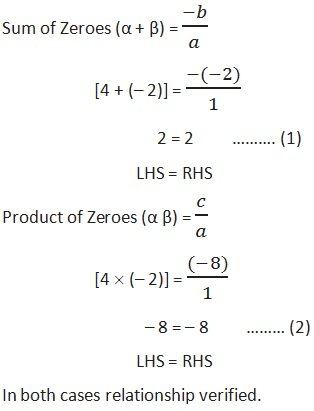Study Materials

# NCERT Solutions for Class 10th Mathematics

Page 2 of 4

## Chapter 2. Polynomials

### Exercise 2.2

Chapter 2. Polynomials

Exercise 2.2

Q1. Find the zeroes of the following quadratic polynomials and verify the relationship between the zeroes and the coefficients.

(i) x2 – 2x – 8

Solution:

Using Middle term splitting method:

x2 – 4x + 2x – 8 = 0

x(x – 4) + 2(x – 4) = 0

x(x – 4) + 2(x – 4) = 0

(x – 4) (x + 2) = 0

x – 4 = 0 x + 2 = 0

x = 4, x = – 2

Zeroes;  α = 4  β = – 2

Verifying the relationship between the zeroes and the coefficients.

a = 1, b = – 2, and c = – 8(ii) 4s2 – 4s + 1

(iii) 6x2 – 3 – 7x

(iv) 4u2 + 8u

(v) t2 – 15

(vi) 3x2x – 4

Q2. . Find a quadratic polynomial each with the given numbers as the sum and product of its zeroes respectively.

Page 2 of 4

Chapter Contents: